# Wishart distribution

The joint distribution of the elements from the sample covariance matrix of observations from a multivariate normal distribution. Let the results of observations have a-dimensional normal distribution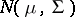with vector meanand covariance matrix. Then the joint density of the elements of the matrix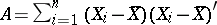is given by the formula(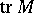denotes the trace of a matrix), if the matrixis positive definite, andin other cases. The Wishart distribution withdegrees of freedom and with matrix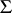is defined as the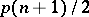-dimensional distributionwith density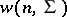. The sample covariance matrix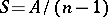, which is an estimator for the matrix, has a Wishart distribution.
The Wishart distribution is a basic distribution in multivariate statistical analysis; it is the-dimensional generalization (in the sense above) of the-dimensional "chi-squared" distribution.
If the independent random vectorsandhave Wishart distributions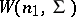and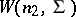, respectively, then the vector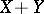has the Wishart distribution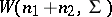.